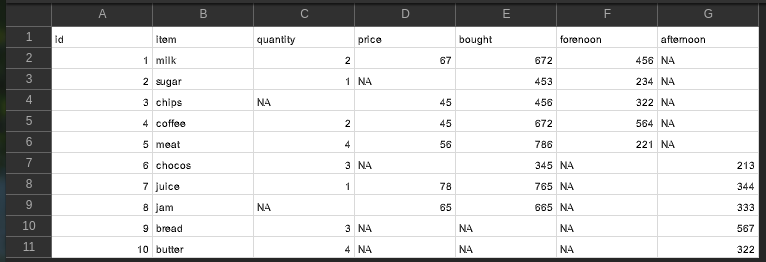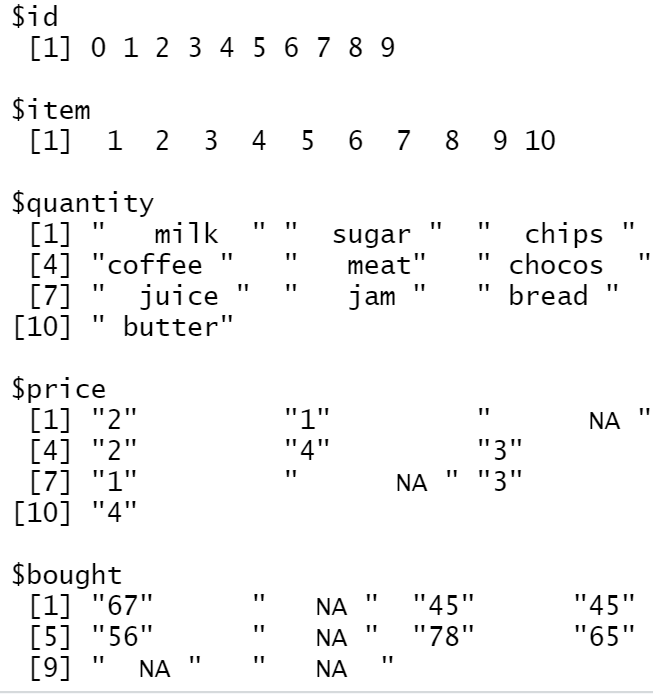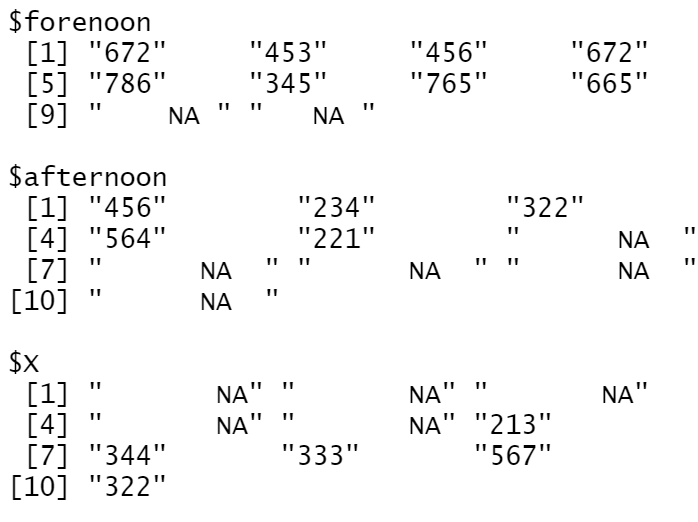Convert CSV to list in R

• Last Updated : 16 May, 2021

In this article, we will discuss how to convert the content of the CSV file to list in R Programming Language.

CSV Used:Method 1 : Using for loop

In this method, the file is first read into the R program and then one by one using for loop the columns are extracted and converted to list explicitly using list() function.

Example :

R

 df=read.csv("item.csv")  lst1=list()    for(i in 1:ncol(df)) {        lst1[[i]] <- df[ , i]    }  names(lst1)=colnames(df)  print(lst1)

Output :Method 2 : Extracting columns separately and pass to list( ) function

In this method, the file is first read and then each column is extracted one by one explicitly with the name of the column. Then using the list method, all the extracted columns are converted to a list using list() in one go. Here names of the extracted columns is given as comma-separated values to list() to get the required functionality.

Example :

R